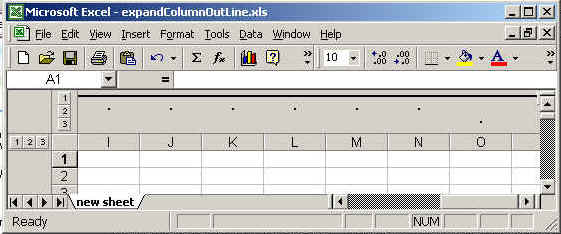# expand the row out line

In this program we are going to create a sheet then create out line for rows and columns. Finally we expand the row outline.# expand the row out line

In this program we are going to create a sheet  then create out line for rows and columns. Finally we expand the row outline.

Code description
The package we need to import is java.io.*,java.util.* ,org.apache.poi.hssf.usermodel.HSSFSheet, and org.apache.poi.hssf.usermodel. HSSFWorkbook.
The method used in this example shift row

grouprow
( startIndex, endIndex):
This is used to create outline for rows startIndex and endIndex shows the starting point and end points for the outline.

groupColumn(startIndex, endIndex):
This is used to create outline for column.

setRowGroupCollapsed(int row, boolean collapse)
This method is used to collapse the row with it value.

You can create the out line for both rows and columns. The out line is shown in output between + and - linked with state line .Then at last we expand the row by using the method setRowGroupCollapsed(int row, boolean collapse). The row value is the number of rows to be expands.

The code of the program is given below:

 ``` <%@ page import="org.apache.poi.hssf.usermodel.HSSFSheet"%> <%@ page import="org.apache.poi.hssf.usermodel.HSSFWorkbook"%> <%@ page contentType="application/vnd.ms-excel" %> <%@ page import="java.io.*" %> <%@ page import="java.util.*" %> <% try{   HSSFWorkbook hwb = new HSSFWorkbook();   HSSFSheet sheet = hwb.createSheet("new sheet");   sheet.groupRow( 4, 10 );   sheet.groupRow( 5, 16);   sheet.groupRow( 13, 15 );   sheet.groupColumn( (short)4, (short)6 );   sheet.groupColumn( (short)5, (short)14 );   sheet.groupColumn( (short)14, (short)14 );     sheet.setRowGroupCollapsed(5, true );   FileOutputStream fileOut = new FileOutputStream ("c://excel//expandRowOutLine.xls");   hwb.write(fileOut);   fileOut.close();     out.println("Your excel file has been generated");      } catch ( Exception ex ) {      }%>```

The output of the program is given below: